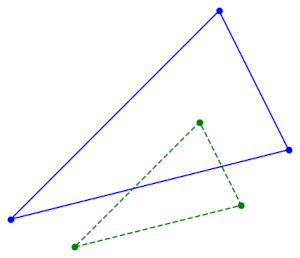# Similar triangles and complex numbersSuppose the vertices of two triangles are given by complex numbers a, b, c and x, y, z. The two triangles are similar ifThis can be found in G. H. Hardy’s classic A Course of Pure Mathematics. It’s on page 93 in the 10th edition.

## Corollary

The theorem above generalizes a result from an earlier post giving a test for whether points determine an equilateral triangle. A triangle ABC is equilateral if it is similar to BCA. For triangles to be similar, corresponding vertex angles must be equal. If you can rotate the order of the angles in one triangle and the two triangles remain similar, the angles must all be equal.

## Proof

Hardy’s proof of the equation above is direct and terse. I’ll quote it here in its entirety.

The condition required is that(large letters denoting the points whose arguments are the corresponding small letters), orwhich is the same as the condition given.

## Commentary

To expand on this a little bit, Hardy is saying that we need to show that if we take two corresponding vertices, A and X, the ratio of the lengths of corresponding sides is the same in both triangles. If memory serves, this is called the “SAS postulate.”

The determinant condition implies the last equation in Hardy’s proof. This is not obvious, but it’s easy to work out with pencil and paper. The reader may be left thinking “OK, I can see it’s true, but why would anyone think of that?” A more motivated derivation would require more background than the reader of the book is presumed to have.

You might object that Hardy’s last equation is a ratio of complex numbers, not a ratio of lengths. But you can take the absolute value of both sides. And the absolute value of a fraction is the absolute value of the numerator divided by the absolute value of the denominator. And now you’re taking a ratio of side lengths. In symbols,